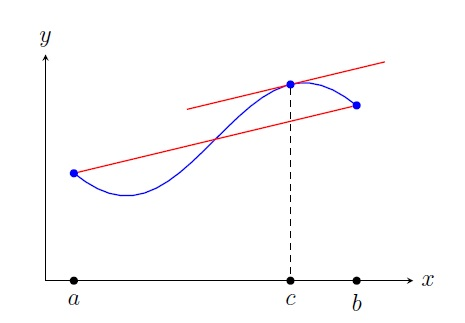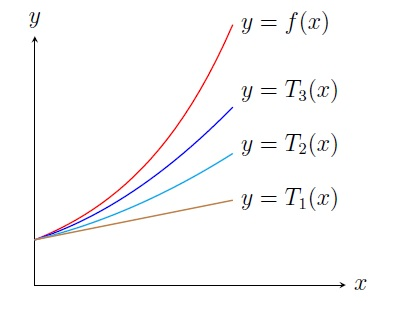Numerical Analysis Home

## Calculus Review

You should revise the limit definitions of the derivative and the Riemann integral of a function from a standard text book. The following are some theorems which will be used later.

Theorem. Let $$f$$ be a differentiable function on $$[a,b]$$.

• $$f$$ is increasing on $$[a,b]$$ if and only if $$f'(x)>0$$ for all $$x\in [a,b]$$.

• If $$f$$ has a local maximum or minimum value at $$c$$, then $$f'(c)=0$$ ($$c$$ is a critical number).

• If $$f'(c)=0$$ and $$f''(c)<0$$, then $$f(c)$$ is a local maximum value.

• If $$f'(c)=0$$ and $$f''(c)>0$$, then $$f(c)$$ is a local minimum value.

Theorem. Let $$f$$ be a continuous function on $$[a,b]$$. If $$f(c)$$ is the absolute maximum or minimum value of $$f$$ on $$[a,b]$$, then either $$f'(c)$$ does not exist or $$f'(c)=0$$ or $$c=a$$ or $$c=b$$.

Intermediate Value Theorem: Let $$f$$ be a function such that
1. $$f$$ is continuous on $$[a,b]$$, and

2. $$N$$ is a number between $$f(a)$$ and $$f(b)$$.
Then there is at least one number $$c$$ in $$(a,b)$$ such that $$f(c)=N$$.

In the particular case when $$f(a)f(b)<0$$, i.e., $$f(a)$$ and $$f(b)$$ are of opposite signs, there is at least one root $$c$$ of $$f$$ in $$(a,b)$$.

Mean Value Theorem: Let $$f$$ be a function such that
1. $$f$$ is continuous on $$[a,b]$$, and

2. $$f$$ is differentiable on $$(a,b)$$.
Then there is a number $$c$$ in $$(a,b)$$ such that $\frac{f(b)-f(a)}{b-a}=f'(c).$Taylor's Theorem: Let $$n\geq 1$$ be an integer and $$f$$ be a function such that
1. $$f^{(n)}$$ is continuous on $$[a,b]$$, and

2. $$f^{(n)}$$ is differentiable on $$(a,b)$$.
Let $$c$$ be a number in $$(a,b)$$. Then for all $$x$$ in $$[a,b]$$, we have $f(x)=f(c)+\frac{f'(c)}{1!}(x-c)+\frac{f''(c)}{2!}(x-c)^2+\cdots +\frac{f^{(n)}(c)}{n!}(x-c)^n +\frac{f^{(n+1)}(\xi)}{(n+1)!}(x-c)^{n+1},$ for some number $$\xi$$ (depends on $$x$$ ) between $$c$$ and $$x$$.Sometimes we simply write $$f(x)=T_n(x)+R_n(x)$$, where $$T_n(x)=\displaystyle\sum_{k=0}^n \frac{f^{(k)}(c)}{k!}(x-c)^k$$ is the Taylor polynomial of degree $$n$$ and $$R_n(x)=\displaystyle\frac{f^{(n+1)}(\xi)}{(n+1)!}(x-c)^{n+1}$$ is the remainder term.

Last edited